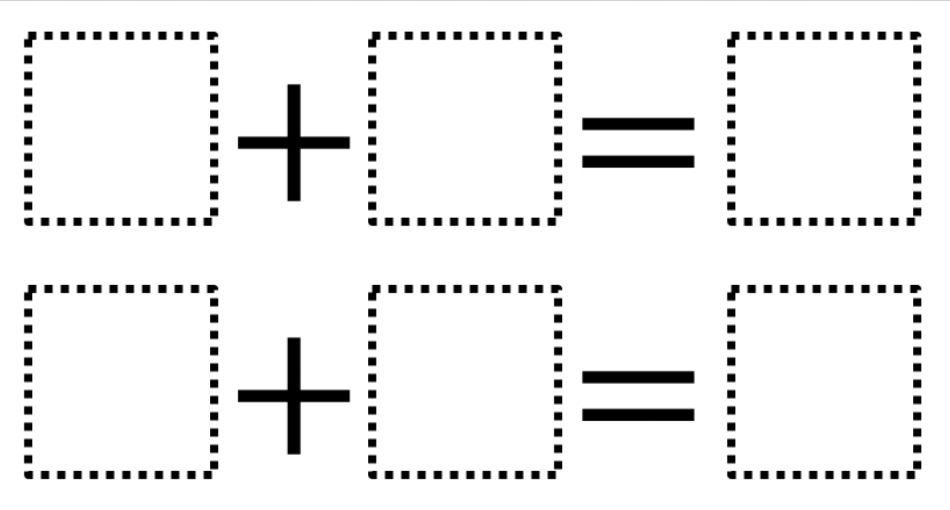Directions: Using the digits 0 to 9 at most one time each, place a digit in each box to create a true statement.### Hint

Can you show two numbers you could add together using your fingers?

There are many answers. Here are some possibilities:
1 + 2 = 3 and 4 + 5 = 9; 2 + 4 = 6 and 5 + 3 = 8.

Source: Stephen Caviness

### Check Also

Directions: Use the digits 1-9, at most once, to complete the equation. What is the …

1.1+2=3
4+5=9

1+3=4
2+5=7

1+4=5
2+6=8

1+5=6
2+3=5

1+6=7
3+5=8

1+7=8
3+6=9

1+8=9
4+3=7

•2+7=9
NOT 2+3=5